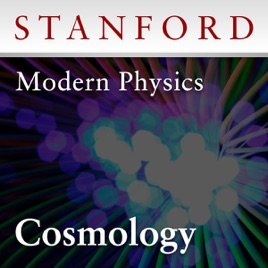10 episodes

The Winter 2013 quarter course of the Modern Physics series concentrates on cosmology, the science of the origin and development of the universe. Along the way, Professor Susskind takes a close look at the Big Bang, the geometry of space-time, inflationary cosmology, cosmic microwave background, dark matter, dark energy, the anthropic principle, and the string theory landscape. To appreciate the material covered in this course, students should understand basic calculus and algebra.# Modern Physics: Cosmology (Winter 2013) Stanford

• Science

The Winter 2013 quarter course of the Modern Physics series concentrates on cosmology, the science of the origin and development of the universe. Along the way, Professor Susskind takes a close look at the Big Bang, the geometry of space-time, inflationary cosmology, cosmic microwave background, dark matter, dark energy, the anthropic principle, and the string theory landscape. To appreciate the material covered in this course, students should understand basic calculus and algebra.

• video
1. Cosmology Lecture 1 (January 14, 2013)

## 1. Cosmology Lecture 1 (January 14, 2013)

Leonard Susskind introduces the study of Cosmology and derives the classical physics formulas that describe our expanding universe. (January 14, 2013)

• video
2. Cosmology Lecture 2 (January 21, 2013)

## 2. Cosmology Lecture 2 (January 21, 2013)

Leonard Susskind solves the expansion equation for universes with zero total energy, and then adds a non-zero total energy term, which leads to an exploration of matter versus radiation dominated universes. (January 21, 2013)

• video
3. Cosmology Lecture 3 (January 28, 2013)

## 3. Cosmology Lecture 3 (January 28, 2013)

Leonard Susskind presents three possible geometries of homogeneous space: flat, spherical, and hyperbolic, and develops the metric for these spatial geometries in spherical coordinates. (January 28, 2013)

• video
4. Cosmology Lecture 4 (February 4, 2013)

## 4. Cosmology Lecture 4 (February 4, 2013)

Leonard Susskind introduces the Einstein field equations of general relativity and thermodynamic equations of state to the analysis of the expanding universe. (February 4, 2013)

• video
5. Cosmology Lecture 5 (February 11, 2013)

## 5. Cosmology Lecture 5 (February 11, 2013)

After reviewing the cosmological equations of state, Leonard Susskind introduces the concept of vacuum energy. Vacuum energy is represented by the cosmological constant, and is also known as dark energy. (February 11, 2013)

• video
6. Cosmology Lecture 6 (February 18, 2013)

## 6. Cosmology Lecture 6 (February 18, 2013)

Leonard Susskind develops the energy density allocation equation, and describes the historical progress of the solution to this equation. He then describes the observations of luminosity and red-shift that have led to the correct solution for today's universe - which is dominated by dark energy.